# Convolutional Neural Network: theory and code

An introductory look at Convolutional Neural Network with theory and code example.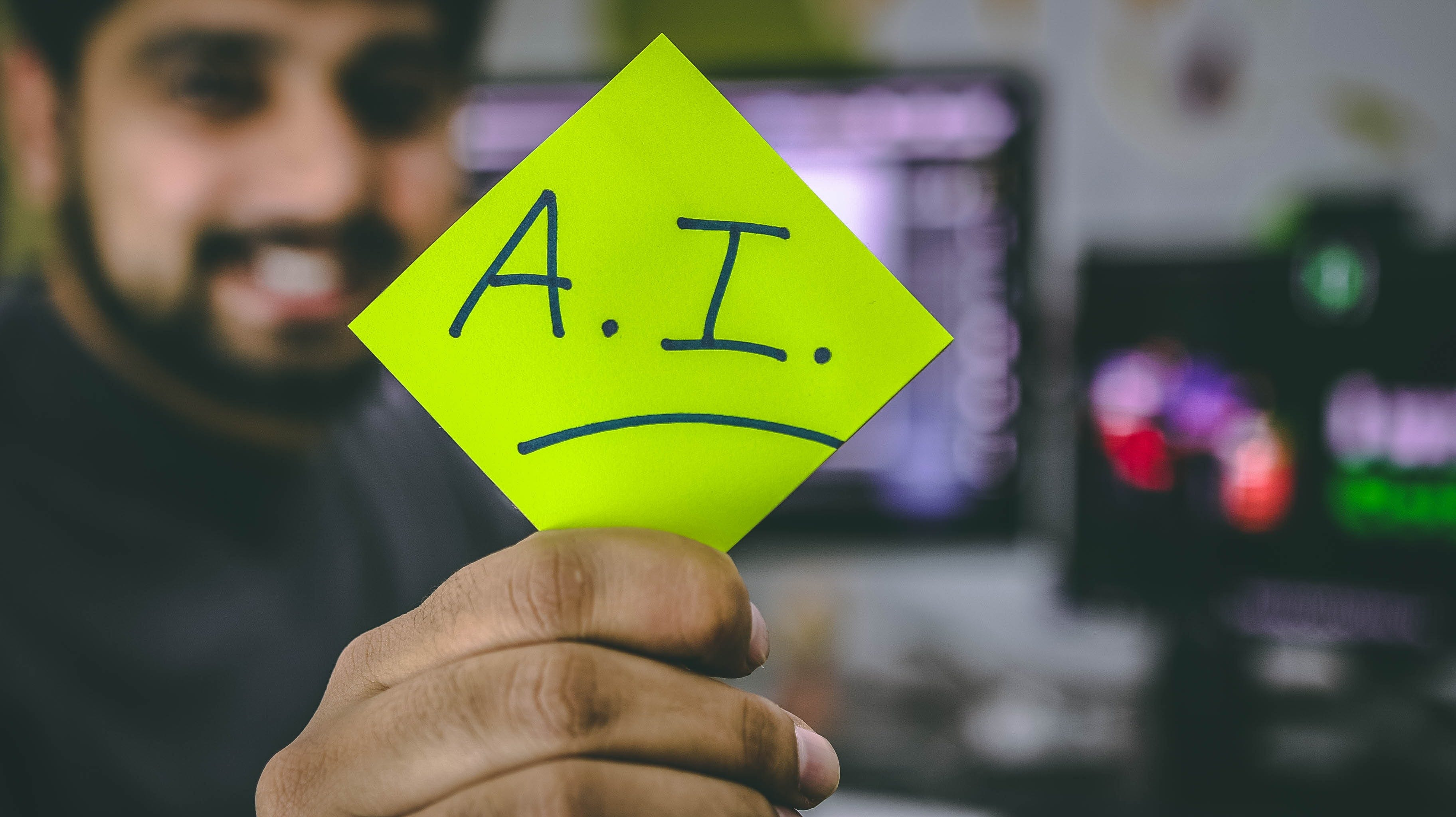Photo by Hitesh Choudhary on Unsplash

I want to write about one of the most important neural networks used in the field of deep learning, especially for image recognition and natural language processing: convolutional neural network, also called “CNN” or “ConvNet”.

TLDR; I divided this article in 2 sections. The first section is about the theory behind the CNN, the second one contains a code example, so if you aren’t interest in theory you can skip section 1. But, honestly, if you understand “just a little” the idea behind the CNN, you will be able to use it more consciously.

Important note: this article is not a “from 0 to 100”, so i’ll take for granted some basic concepts about AI, likes feedforward neural networks, activation functions and so on.

# Section 1: theory

What is a convolutional neural network and why use it?

Before answering to this question, we need to take a step back and consider a feedforward neural network. This architecture was one of the first and simplest networks built. The basic idea is that each neuron is connected with all the other neurons (there are no loops in the network), where the informations move only forward starting from the input layer, through the hidden layers, to the output layer.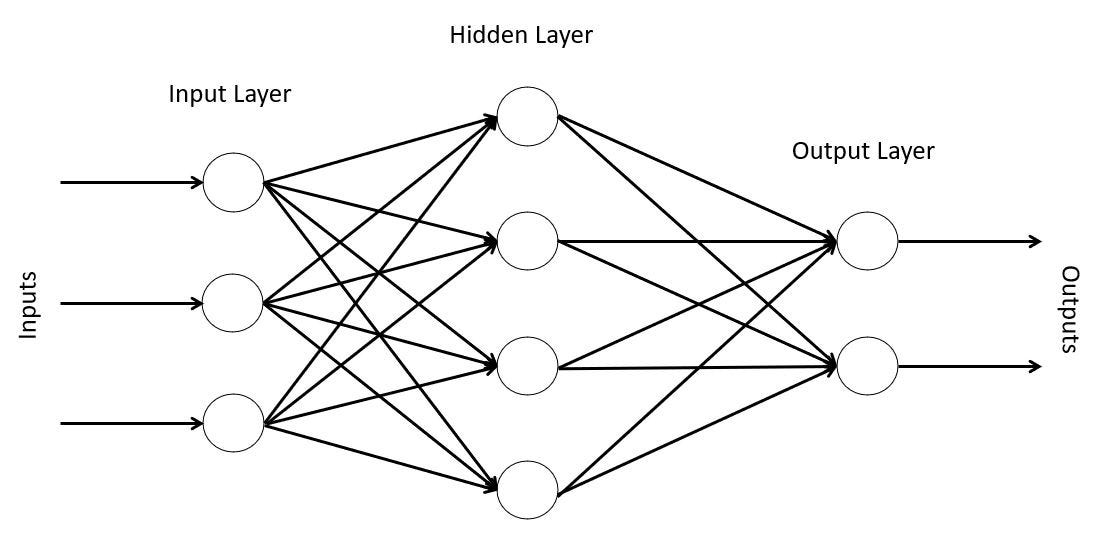Feed Forward

This network works well in many cases, but one of the weaknesses concerns the computer vision. If we want to analyze some complex images, the feed forward is unable to extract the most importants informations from it, like the subjects in a photo.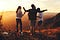Is a feed forward network able to recognize the subjects in this photo? (Photo by Helena Lopes on Unsplash)

The convolutional neural networks were created to solve this problem: basically, a CNN takes in input an image and analyzes it, so it’s able to classify the objects present in it. This means that a CNN is able to capture the spatial informations of an image.

How does a CNN work?

Here’s a picture showing how a CNN works: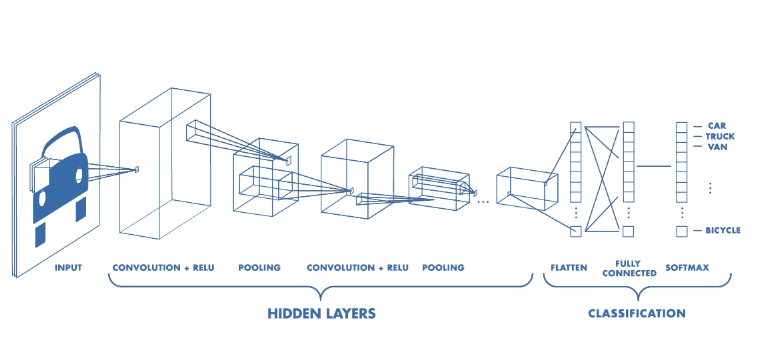source

Intuitively, the network is composed by an input layer, an output layer, and by one or more hidden layers (convolution and pooling), whose goal is to capture the most relevant informations from an image.

Of course, this brief introduction isn’t enough to explain how CNN works, so let’s take a deeper look.

Input

As I wrote before, the input of a CNN is an image.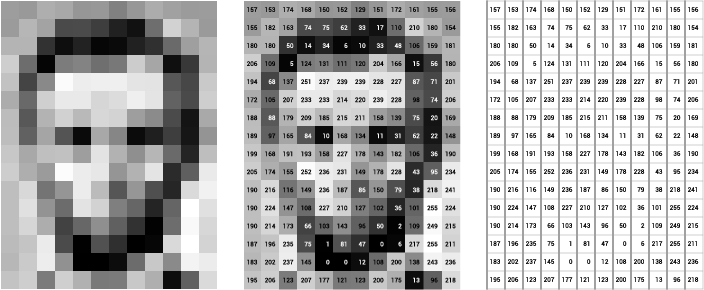source

A greyscale image is presented by pixels matrix, where each pixel correspond to a value from 0 (black pixel) to 255 (white pixel). Since the primary color of this image is grey, that means it has 1 channel. This information will be useful later (for more click here).

A color image (RGB) is presented by three pixels matrices, one for each primary color (red, green and blue). Since the primary colors of a RGB image are red, green and blue, that means it has 3 channels.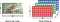source

Basically, we can say that a CNN takes in input a pixels matrix (the image) with n channels, where n is the number of the primary colors.

Convolutional Layers

These layers are called so because they perform a convolution operation. Briefly:

convolution is a mathematical operation on two functions that produces a third function expressing how the shape of one is modified by the other.

How this operation is applied to a CNN? Let’s suppose we have in input a greyscale image, and the correspondant matrix is the follow: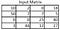Input matrix

Now we must define a “convolution matrix” named as filter or kernel, whose main goal is to apply a filter to the image. Applying the kernel to the input matrix allows to highlight its characteristics, such as the edges, the subjects and so on. Having said that, let’s define the kernel as follow: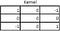Kernel “Edge-detect”

and now let’s see the convolution operation in action: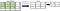First step

The first step is to take from the input matrix a submatrix (let’s call it “A”) of the same size as kernel starting from position (1,1) and multiply A and kernel. The mathematical operation is:

(10 x 1)+(2 x 0)+(0 x -1)+(50 x 0)+(7 x 0)+(7 x 0)+(0 x -1)+(0 x 0)+(25 x 1)=35

then we move one position to the right and repeat the operation: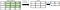Step 2

since we are “at the farest right” of the input matrix, we move one position lower and repeat the operation again: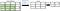Step 3

last step: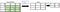Step 4

Done! This output matrix (called feature map) has two characteristics:

1. the dimension is lower than the input matrix (from 4 x 4 to 2 x 2);
2. the values ​​inside highlight the characteristics of the starting image (like the edges).

We can notice that we started the operation from the top-left corner of matrix and we slided to the right of one position, until we reached the top-right corner. Then we moved to the bottom of one position and we repeated the process again, until the input matrix is examinated.

And that’s all about convolution. Next step: pooling layer.

Pooling layers

The convolutional layers are very effective to find important features from images. Hovewer, there is one limitation:

A limitation of the feature map output of convolutional layers is that they record the precise position of features in the input. This means that small movements in the position of the feature in the input image will result in a different feature map. This can happen with re-cropping, rotation, shifting, and other minor changes to the input image.

(Click here to read the full article.)

This is when the pooling layers “come to play”, that are commonly applied after a convolution layer. There are two types of pooling:

Max pooling

An operation that selects the maximum value in every specific region of the feature map. Example: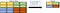Max pooling with stride (2, 2)

Average pooling

An operation that selects the average value in every specific region of the feature map. Example: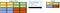Average pooling with stride (2, 2)

The pooling layers are useful especially because they reduce the dimension of feature maps (therefore the computational cost decreases) and at the same time they extract the most important informations, making the model more robust to noise.

Classification: fully connected layers

Here we are into the final step of creating a CNN: we add fully connected layers as final layers of the model in order to have a better accuracy in classifiyng the images. Before doing that, we must “adjust” the dimensions, because in the previous layers (convolution and pooling) we worked with matrices, but the fully connected layers take in input a vector. To do so, we need to “flat” the matrix into a vector, like in the following image: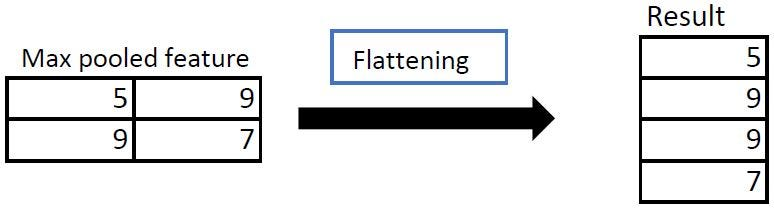Flatten operation

In the image above we take the result matrix with dimensions (2, 2) obtained from the max pooling operation and we “flat” it into a vector of dimension (4,1). Once this operation is completed, we can proceed with the insertion of one or more fully-connected layers in order to improve the classification!

And that’s all about the theory behind the Convolutional Neural Network! In the next section we will implement the code of a CNN.

# Section 2: Code

In this section we explore an example code. The dataset used is the fashion MNIST dataset:

is a dataset of Zalando’s article images — consisting of a training set of 60,000 examples and a test set of 10,000 examples. Each example is a 28x28 grayscale image, associated with a label from 10 classes.

The labels are the following:

So, let’s get started!

Import libraries and load dataset

First we need to import all the libraries.

`import tensorflow as tffrom tensorflow import kerasimport numpy as npimport matplotlib.pyplot as pltfrom tensorflow.keras import datasets, layers, models`

Let’s download the Fashion MNIST dataset.

`fashion_mnist = keras.datasets.fashion_mnist(train_images, train_labels), (test_images, test_labels) = fashion_mnist.load_data()train_images.shape(60000, 28, 28)`

The training set has 60000 images, each one is a 28x28 greyscale image that has associated a label from 0 to 9 (included).

`test_images.shape(10000, 28, 28)`

The test set has 10000 images.

Explore and prepare the dataset for CNN

It’s always an excellent idea to explore the dataset before creating a neural network, so let’s take a look at 15 images from training set:

`plt.figure(figsize=(10,10))class_names = ['T-shirt/top', 'Trouser', 'Pullover', 'Dress', 'Coat','Sandal', 'Shirt', 'Sneaker', 'Bag', 'Ankle boot']for i in range(15):    plt.subplot(5,5,i+1)    plt.xticks([])    plt.yticks([])    plt.grid(False)    plt.imshow(train_images[i], cmap=plt.cm.binary)    plt.xlabel(class_names[train_labels[i]])plt.show()`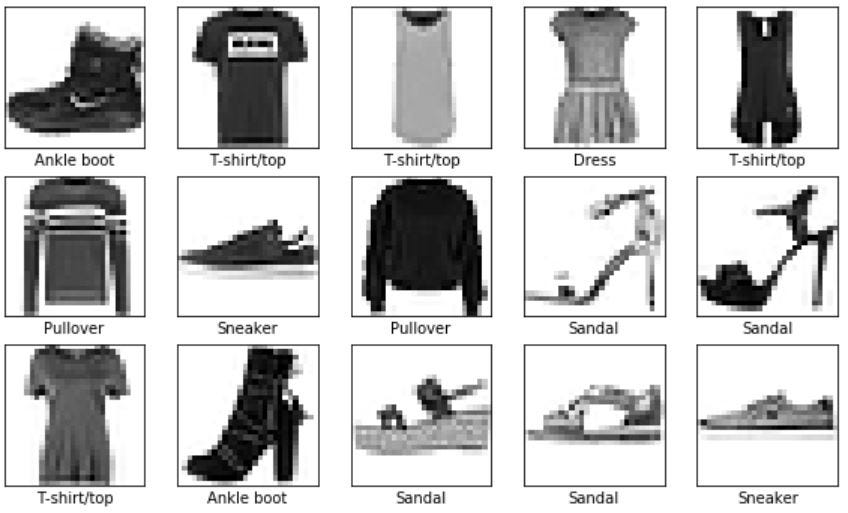15 images from training set

Since the images have values from 0 (black pixel) to 255 (white pixel), we need to scale it from 0 to 1. This will be useful for the CNN.

`train_images = train_images / 255.0test_images = test_images / 255.0`

The last step is to adjust the shape of training and test set. What does it mean?

`train_images.shape(60000, 28, 28)`

As we can see in the cell above, the training set have 60000 images, each one is 28x28 pixels matrix. But in “train_images.shape” is missing the number of channels, so we need to specify it. Obviously, our images are in greyscale, so the number of channels is 1.

`# reshape in training settrain_images = train_images.reshape((train_images.shape, 28, 28, 1))# reshape in test settest_images = test_images.reshape((test_images.shape, 28, 28, 1))#### new shape of train and test set ####train_images.shape, test_images.shape((60000, 28, 28, 1), (10000, 28, 28, 1))`

Perfect! Now the shape of training and test set is correct!

Building the CNN

Finally we can proceed to build the CNN. We create it step by step, adding one layer at time.

`# create an empty model (sequential)model = models.Sequential()# add first 2D convolution Layermodel.add(layers.Conv2D(filters=32, kernel_size=(3, 3), padding="same", strides=1, activation='relu', input_shape=(28, 28, 1)))`

First thing to say, we use a convolution layer 2D because we are working with images. Second, there are a lot of arguments here! Let’s examine it:

1. filters = 32 → the number of kernels (or filters) to use in the convolution operation. Each of these operations produce a feature map. Generally, more filters produce more feature map with different properties in prominence.
2. kernel_size = (3, 3) → the dimension of kernels. In our case, the kernels dimension will be 3x3.
3. padding = “same” → as I wrote before, when we apply a convolution operation, we obtain a new matrix called “feature map”. This matrix has a lower dimension if compared with the input matrix. With the argument “same”, we keep the same dimension of the input matrix (28 x 28 pixels). In our case this will help us to improve the accuracy, but this is not always true!
4. strides = 1 → in the convolution operation we take a submatrix from an input matrix, we multiply it with the kernel and then we move to the right of one position, and so on. This argument let us choose of how many positions we want to slide after each multiplication. (Default is 1).
5. activation = “relu” → the activation function used in convolution layer.
6. input_shape=(28, 28, 1) → this is a tuple, containing the dimension of matrix (28 x 28 pixels) and the number of channels. NOTE: we don’t need to specify the number of the images in training set (=60000), the model will “take care” of it by itself.

Ok, now it’s time for max pooling layer!

`model.add(layers.MaxPooling2D(pool_size=(2, 2)))`

I already explained the max pooling operation. The argument “pool_size” is just the matrix dimension for the pooling operation.

Let’s add more Conv and pooling layers!

`model.add(layers.Conv2D(filters=64, kernel_size=(3, 3), padding="same", strides=1, activation='relu'))model.add(layers.MaxPooling2D((2, 2)))model.add(layers.Conv2D(filters=64, kernel_size=(3, 3), padding="same", strides=1, activation='relu'))`

Last thing to do is to add the fully-connected layers:

`model.add(layers.Flatten())model.add(layers.Dense(units=64, activation='relu'))model.add(layers.Dense(10))`

The flatten layer just flattens the input from the previous layers. A dense layer with 64 neurons improve further the model accuracy. The final layer is the output layer, I choose 10 neurons because we have 10 labels (T-shirt, pullover and so on).

Model summary

Now it’s time to explore the summary of the model and see the layers Output Shape:

`model.summary()Model: "sequential"_________________________________________________________________Layer (type)                 Output Shape              Param #   =================================================================conv2d (Conv2D)              (None, 28, 28, 32)        320       _________________________________________________________________max_pooling2d (MaxPooling2D) (None, 14, 14, 32)        0         _________________________________________________________________conv2d_1 (Conv2D)            (None, 14, 14, 64)        18496     _________________________________________________________________max_pooling2d_1 (MaxPooling2 (None, 7, 7, 64)          0         _________________________________________________________________conv2d_2 (Conv2D)            (None, 7, 7, 64)          36928     _________________________________________________________________flatten (Flatten)            (None, 3136)              0         _________________________________________________________________dense (Dense)                (None, 64)                200768    _________________________________________________________________dense_1 (Dense)              (None, 10)                650       =================================================================Total params: 257,162Trainable params: 257,162Non-trainable params: 0_________________________________________________________________`
• Layer 1 “conv2d”: (None, 28, 28, 32) → the “None” value refers to the batch size, because the model doesn’t know it before the training. The values “28, 28” refer to the matrix output dimension. Since we choosen “padding=same”, the dimension will be the same as the input matrix. The last value “32” is the number of matrices after the convolution operation.
• Layer 2 “max_pooling2d”: (None, 14, 14, 32) → the first 2 values (excluding None) are the matrix output dimension after the max pooling operation. Since we choosen the pooling size=“(2,2)” the output will be (14, 14). Again, 32 is the number of matrices after the pooling operation.

The same logic is applied also for layers “conv2d_1”, “max_pooling2d_1” and “conv2d_2”. The last relevant thing to say it’s about the output shape of flatten layer. This layer just take the output shape of the previous layer, (7, 7, 64) and it flattens it, multiplying the values, so, 7x7x64=3136.

Train the model

Now it’s time to train the model:

`model.compile(optimizer='adam',              loss=tf.keras.losses.SparseCategoricalCrossentropy(from_logits=True), metrics=['accuracy'])history = model.fit(train_images, train_labels, epochs=10,                     validation_data=(test_images, test_labels))`

We use the loss function SparseCategoricalCrossentropy, as reported in tensorflow documentation: “Computes the crossentropy loss between the labels and predictions”. We train the model for 10 epochs.

Let’s see the training of the model:

`Epoch 1/101875/1875 [==============================] - 55s 29ms/step - loss: 0.4014 - accuracy: 0.8549 - val_loss: 0.2938 - val_accuracy: 0.8946Epoch 2/101875/1875 [==============================] - 58s 31ms/step - loss: 0.2582 - accuracy: 0.9064 - val_loss: 0.2718 - val_accuracy: 0.9021[...]Epoch 9/101875/1875 [==============================] - 57s 30ms/step - loss: 0.0889 - accuracy: 0.9660 - val_loss: 0.2936 - val_accuracy: 0.9179Epoch 10/101875/1875 [==============================] - 55s 29ms/step - loss: 0.0738 - accuracy: 0.9721 - val_loss: 0.3055 - val_accuracy: 0.9225`

In the next subsection we explore the results.

Results evaluation

The final step of this guide is to evaluate the results of CNN.

`test_loss, test_acc = model.evaluate(test_images, test_labels, verbose=2)print('\nTest accuracy:', test_acc)313/313 - 2s - loss: 0.3055 - accuracy: 0.9225`

We obtained a test loss of 0.3055 and a test accuracy of 0.9225. Not bad! However there are models that have a better accuracy (this is not the main focus of this guide).

Last thing, let’s examine the accuracy and loss over the epochs:

`plt.plot(history.history['accuracy'], label='accuracy')plt.plot(history.history['val_accuracy'], label = 'val_accuracy')plt.xlabel('Epoch')plt.ylabel('Accuracy')plt.ylim([0.5, 1])plt.legend(loc='lower right')test_loss, test_acc = model.evaluate(test_images,  test_labels, verbose=2)`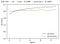Accuracy Chart

This chart shows the training accuracy (blue line) and the validation accuracy (orange line). We can notice that over the epochs the accuracy slowly increases, until the last epoch where it reaches the value of 92%. Also, the training accuracy is higher than validation accuracy, that means there is overfitting; if we want, we can solve the problem just adding a dropout layer.

And that’s all for this tutorial, thanks for readintg! I hope it was useful!